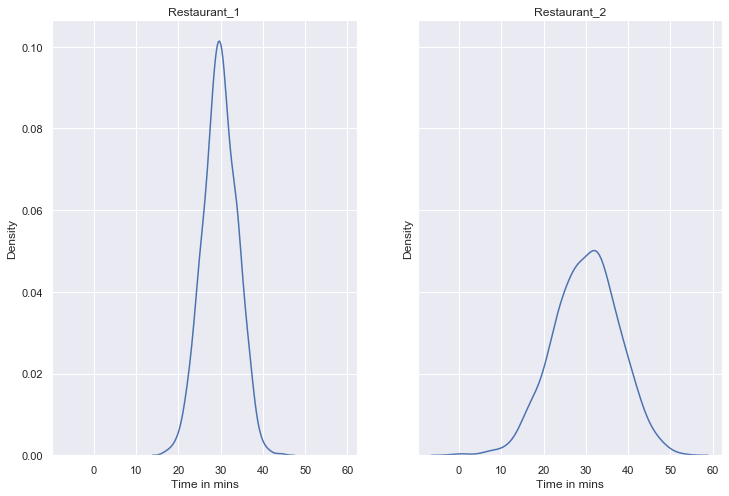Search
•SoniaSamipillai

# Descriptive Statistics - Measures of Dispersion

Updated: Jan 25, 2021The goal is to turn data into information, and information into insight.

Measures of Variability helps determine the extent to which a distribution is stretched or squeezed. While a measure of central tendency describes the typical value, measures of variability define how far away the data points tend to fall from the center. Measures of variability are often considered in addition to the Measures of Location to consider the dispersion. A low dispersion indicates that the data points tend to be clustered tightly around the center. High dispersion signifies that they tend to fall further away.

For example, Say suppose you like to order food from two restaurants that equally provide great tasty food. The mean number of hours to fill their orders is 30 mins for both the restaurants. Note that this is the average number. We do not know the variability in the number of hours. Let us look at the dispersion of delivery data collected over a month on a daily basis for your area.Comparing the variabilities between the two restaurants, we can see though they have the same mean, the distribution of values around the mean are different. When the variability is less the results are more consistent and dependable and less uncertainty. Definitely I will place my order at restaurant 1 looking at the variability of the data. This explains why variability is an important measure to consider. The code for the above plot is below.

So now you know why you cannot solely depend on the measures of location. We also need to know the dispersion of data.

The most common measures of variability are:

1. Range

2. Interquartile Range

3. Standard Deviation

4. Variance

5. Coefficient of Variation

Range

Range is the simplest measure of variability. Range is a measure that can give you an idea about dispersion. However Range is affected by outliers.Interquartile Range

A measure of variability that overcomes the dependency on extreme values is the InterQuartile Range,IQR. This measure of variability is the difference between the third quartile, Q3 and first quartile, Q1.Variance

The variance is a measure of variability that is based on the difference between the value of each observation(xi) and the mean. The difference between each xi and the mean (xbar for a sample and mu for a population) is called a deviation about the mean. In the computation of variance, the deviations about the mean are squared. A measure of variability based on the squared deviations of the data values about the mean.Standard Deviation

A measure of variability computed by taking the positive square root of the variance. The sample standard deviation is a point estimator of the population standard deviation. Variance and standard deviation are both of measures of dispersion. Standard deviation is derived from variance.

Why do we need standard deviation when there is variance? The reason behind is that the standard deviation is easier to interpret than variance because the standard deviation is measured in the same units as the data. Because of this, we can easily compare standard deviation with other measures of descriptive statistics.Coefficient of Variation

The coefficient of variation is a relative measure of variability. It measures the standard deviation relative to the mean.

A measure of relative variability computed by dividing the standard deviation by the mean and multiplying by 100.Now that we know the Measures of location and Measures of Variability and why are they important, in the next blog we can discuss about the measure of the shape of a distribution.

Hope this post was helpful!!. If you’re interested to read more, you can subscribe and be notified when the next article is published.